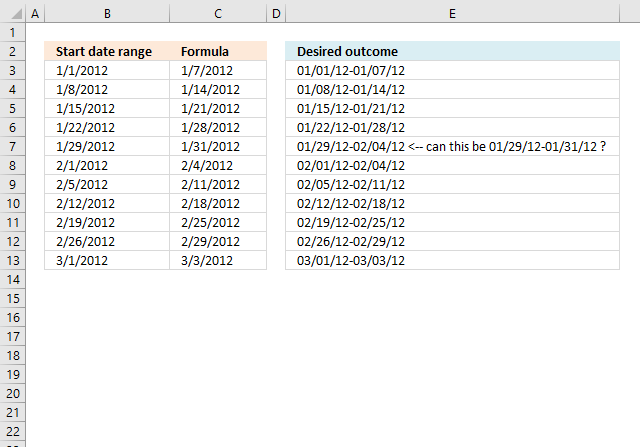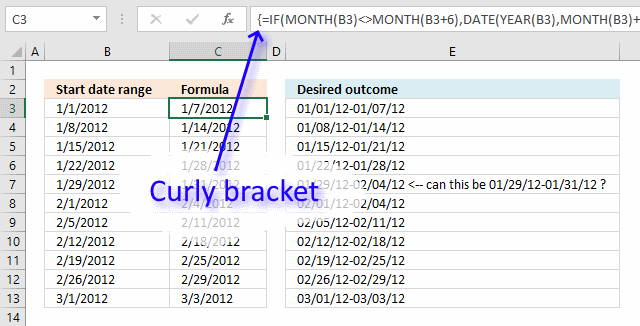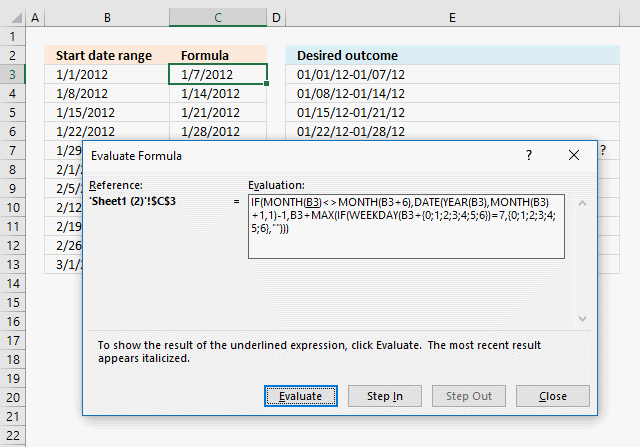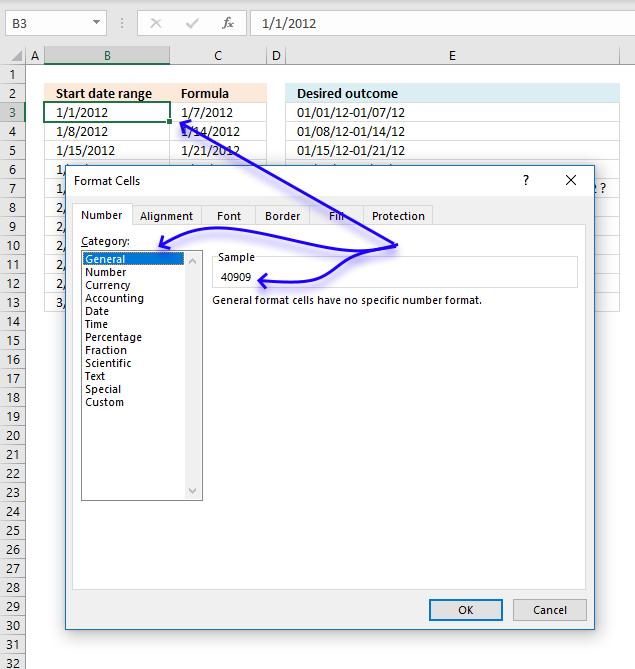Author: Oscar Cronquist Article last updated on August 17, 2019This article demonstrates a formula that creates date ranges based on a given number of days and the end date range falls within the given month.

Column c shows what the formula returns demonstrated in this article Create a date range , however, Anees asks if it is possible to return the last date in the current month so they date range stays within the given month for that particular date range.

Hi,The above formula in A9 "Increasing date in a column" works pretty well however I have a small request to change the formula as per my need.

As I fill the first column in excel with this formula than use + handler on the bottom right of the column to drag and copy it to next cell so the date increases.

That works but when it reaches the end of month it continues in the same cell.

Is it possible so at the end of the month the range would stay within the month instead of increasing to next month?

Here let me try to explain visually.
01/01/12-01/07/12
01/08/12-01/14/12
01/15/12-01/21/12
01/22/12-01/28/12
01/29/12-02/04/12 And next month would be per week in each column as well and so on.
02/01/12-02/04/12
02/05/12-02/11/12
02/12/12-02/18/12
02/19/12-02/25/12
02/26/12-02/29/12
03/01/12-03/03/12

Thank you.

The array formula becomes quickly complicated if I try to concatenate the start and end date in one cell. I am going to use two cells in this example, the start date in one cell and the end date in another cell.

The date in cell B3 is the start date of the first date range, the second date range begins with the next day after the previous date range's end date.

Date in cell B3:

1/1/2012

Array Formula in cell C3:

=IF(MONTH(B3)<>MONTH(B3+6),DATE(YEAR(B3),MONTH(B3)+1,1)-1,B3+MAX(IF(WEEKDAY(B3+{0;1;2;3;4;5;6})=7,{0;1;2;3;4;5;6},"")))

I will explain this formula later in this article, first I'll show you how to enter this formula as an array formula. This is important to make the formula work.

How to create an array formula

1. Copy above array formula.
2. Select cell C3.
3. Paste above array formula to cell.
4. Press and hold Ctrl + Shift simultaneously.
5. Press Enter once.
6. Release all keys.

If you did this right the formula begins with a curly bracket and ends with a curly bracket, do not enter these characters yourself.An array formula allows you to perform multiple calculations simultaneously using multiple cells if needed, this makes Excel really powerful and fun to use. Check out the advanced formulas category for more incredible formulas.

How to copy array formula in cell C3

1. Select cell C3
2. Copy (Ctrl +c)
3. Select cell range C4:C13
4. Paste (Ctrl + v)

It is important that you copy the cell and not the formula, there are relative cell references that need to change accordingly in order for this formula to work as intended.

Formula in cell C4:

=C3+1

This formula is a regular formula, it simply adds 1 to the end date of the previous date range.

Copy cell C4 and paste to cells below as far as needed.

Explaining array formula in cell C3

You can easily follow along using the "Evaluate Formula" feature that you can find on tab "Formulas" on the ribbon.Press with left mouse button on "Evaluate" button to see the formula calculations step by step, this is great if you need to troubleshoot a formula.

Step 1 - Build logical expression

The MONTH function calculates the month as a number. 1 is January, 2 is February and so on. 12 is December. This formula adds 6 to the start date and if that date belongs to the next month we need to know that.

MONTH(B3)<>MONTH(B3+6)

becomes

MONTH(40909)<>MONTH(40909+6)

Excel handles dates as numbers, 1 is 1/1/1900 and 40909 is 1/1/2012. You can verify this by selecting cell C3 and press CTRL + 1 which is the shortcut to open the Format cells dialog box.Press with left mouse button on "General" to see the number that Excel uses as 1/1/2012, then press with left mouse button on "Cancel" button.

MONTH(40909)<>MONTH(40909+6)

becomes

MONTH(40909)<>MONTH(40915)

becomes

1<>1

The less than sign and the greater than sign combined is the same as not equal to.

1<>1 is the same as 1 is not equal to 1 and that returns the boolean value FALSE.

40909 is 1/1/2012 and 40915 is 1/7/2012, both dates are in January. January is 1 and the logical expression returns FALSE.

Step 2 - Compare dates and check if months are not equal

The IF function needs a boolean value in order to determine which part of the formula to calculate. I have simplified the formula, formula_TRUE is evaluated if the logical expression returns TRUE and formula_FALSE is evaluated if the logical expression returns FALSE.

IF(MONTH(B3)<>MONTH(B3+6), formula_TRUE, formula_FALSE)

becomes

IF(FALSE, formula_TRUE, formula_FALSE)

and returns formula_FALSE. formula_FALSE is B3+MAX(IF(WEEKDAY(B3+{0;1;2;3;4;5;6})=7,{0;1;2;3;4;5;6},""))

Step 3 - Calculate days to last day in week

This step is calculated if the logical expression returns FALSE.

B3+MAX(IF(WEEKDAY(B3+{0;1;2;3;4;5;6})=7,{0;1;2;3;4;5;6},""))

becomes

40909+MAX(IF(WEEKDAY(40909+{0, 1, 2, 3, 4, 5, 6})=7, {0, 1, 2, 3, 4, 5, 6}, ""))

becomes

40909+MAX(IF(WEEKDAY({40909, 40910, 40911, 40912, 40913, 40914, 40915})=7, {0, 1, 2, 3, 4, 5, 6}, ""))

becomes

40909+MAX(IF({1, 2, 3, 4, 5, 6, 7}=7, {0, 1, 2, 3, 4, 5, 6}, ""))

becomes

40909+MAX({"", "", "", "", "", "", 6})

becomes

40909+6

and returns 40915 (2012-01-07) in cell A2.

Step 4 - Calculate last day in month

This step is calculated if the logical expression returns TRUE, that happens in cell C7.

IF(MONTH(B7)<>MONTH(B7+6), formula_TRUE, formula_FALSE)

becomes

=IF(MONTH(40937)<>MONTH(40943), formula_TRUE, formula_FALSE)

becomes

=IF(1<>2, formula_TRUE, formula_FALSE)

and returns TRUE.

DATE(YEAR(B7),MONTH(B7)+1,1)-1

becomes

DATE(YEAR(40937), MONTH(40937)+1, 1)-1

becomes

DATE(2012, 2, 1)-1

becomes

40940-1

and returns 40939 in cell C7. (1/31/2012)

Get the Excel fileAnees.xlsx